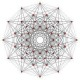The Unapologetic Mathematician

The Interior Product

We have yet another operation on the algebra$\Omega(M)$ of differential forms: the “interior product”. Given a vector field$X\in\mathfrak{X}(M)$ and a$k$-form$\omega\in\Omega^k(M)$, the interior product$\iota_X(\omega)$ is the$k-1$-form defined by$\displaystyle\left[\iota_X\omega\right](X_1,\dots,X_{k-1})=\omega(X,X_1,\dots,X_{k-1})$

That is, we just take the vector field$X$ and stick it into the first “slot” of a$k$-form. We extend this to functions by just defining$\iota_Xf=0$.

Two interior products anticommute:$\iota_X\iota_Y=-\iota_Y\iota_X$, which follows easily from the antisymmetry of differential forms. Each one is also clearly linear, and in fact is also a graded derivation of$\Omega(M)$ with degree -1:$\displaystyle\iota_X(\alpha\wedge\beta)=(\iota_X\alpha)\wedge\beta+(-1)^{-p}\alpha\wedge(\iota_X\beta)$

where$p$ is the degree of$\alpha$. This can be shown by reducing to the case where$\alpha$ and$\beta$ are wedge products of$1$-forms, but rather than go through all that tedious calculation we can think about it like this: sticking$X$ into a slot of$\alpha\wedge\beta$ means either sticking it into a slot of$\alpha$ or into one of$\beta$. In the first case we just get$\iota_x\alpha$, while in the second we have to “move the slot” through all of$\alpha$, which incurs a sign of$(-1)^p=(-1)^{-p}$, as asserted.

July 26, 2011 - Posted by | Differential Topology, Topology

1. Why is this post in the “point-set topology” category?Comment by Andrei | July 26, 2011 | Reply

2. An errant click on a very tightly-spaced list.Comment by John Armstrong | July 26, 2011 | Reply

3. […] turns out that there is a fantastic relationship between the interior product, the exterior derivative, and the Lie […]

Pingback by Cartan’s Formula « The Unapologetic Mathematician | July 26, 2011 | Reply

4. […] along the projection to get a “time” vector field on the cylinder. Then we use the interior product to assert that and […]

Pingback by The Poincaré Lemma (proof) « The Unapologetic Mathematician | December 3, 2011 | Reply

5. […] and applying it to the vector itself: . We also take the canonical volume form , and we use the interior product to define the -form […]

Pingback by A Family of Nontrivial Homology Classes (part 1) « The Unapologetic Mathematician | December 20, 2011 | Reply

6. hi thank you for this post.

when we insert X into a slot of say a, then don’t we need to move X so that it will be on the first slot of a? And this will require multiplying by (-1) times the number of swaps.Comment by circa1687 | November 15, 2015 | Reply

7. That’s true, circa1687. Think of it like this: because of antisymmetry, the interior product doesn’t really care which slot you insert$X$ into, since it just picks up a sign as you move it to the front. Inserting into the second slot of$\alpha$ is the same as inserting it into the second slot of$\alpha\wedge\beta$, but inserting into the second slot of$\beta$ is like inserting it into the$p+2$th slot of$\alpha\wedge\beta$, so we always pick up an extra$p$ signs.Comment by John Armstrong | November 15, 2015 | Reply